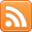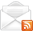# ASP.NET,C#.NET,VB.NET,JQuery,JavaScript,Gridview

aspdotnet-suresh offers C#.net articles and tutorials,csharp dot net,asp.net articles and tutorials,VB.NET Articles,Gridview articles,code examples of asp.net 2.0 /3.5,AJAX,SQL Server Articles,examples of .net technologies

### KeyBoard Event Key Codes in AS3 | Alphabet, Numbers, Special Character Codes

Apr 23, 2013
Introduction:

Here I will explain as3 keyboard event key codes or alphabet character codes or number character codes or special characters key codes or the character code value of the key pressed or released.

Description:

In previous articles I explained create symbols(copyright, trademark etc..) with keyboard, and many articles relating to Internet Tips. Now I will explain character code value of the key pressed or released.

Check below table for character code value of the key pressed or released
 KeyBoard Character Key Codes Backspace = 8 Tab = 9 Enter = 13 Shift = 16 Ctrl = 17 Alt  = 18 CapsLock = 20 Esc = 27 Spacebar = 32 PageUp = 33 PageDown = 34 End = 35 Home = 36 LeftArrow = 37 UpArrow = 38 RightArrow = 39 DownArrow = 40 Insert = 45 Delete = 46 NumLock = 144 ScrLk = 145 Pause/Break = 19 A or a = 65 B or b = 66 C or c = 67 D or d = 68 E or e = 69 F or f = 70 G or g = 71 H or h = 72 I or i = 73 J or j = 74 K or k = 75 L or l = 76 M or m = 77 N or n = 78 O or o = 79 P or p = 80 Q or q = 81 R or r = 82 S or s = 83 T or t = 84 U or u = 85 V or v = 86 W or w = 87 X or x = 88 Y or y = 89 Z or z = 90 0 = 48 1 = 49 2 = 50 3 = 51 4 = 52 5 = 53 6 = 54 7 = 55 8 = 56 9 = 57 ; or : = 186 = or + = 187 - or _ = 189 / or ? = 191 ` or ~ = 192 [ or { = 219 \ or | = 220 ] or } = 221 " or ' = 222 , = 188 . = 190 / = 191 Numpad 0 = 96 Numpad 1 = 97 Numpad 2 = 98 Numpad 3 = 99 Numpad 4 = 100 Numpad 5 = 101 Numpad 6 = 102 Numpad 7 = 103 Numpad 8 = 104 Numpad 9 = 105 Numpad Multiply = 106 Numpad Add = 107 Numpad Enter = 13 Numpad Subtract = 109 Numpad Decimal = 110 Numpad Divide = 111 F1 = 112 F2 = 113 F3 = 114 F4 = 115 F5 = 116 F6 = 117 F7 = 118 F8 = 119 F9 = 120 F10 = no key F11 = 122 F12 = 123 F13 = 124 F14 = 125 F15 = 126

 If you enjoyed this post, please support the blog below. It's FREE! Get the latest Asp.net, C#.net, VB.NET, jQuery, Plugins & Code Snippets for FREE by subscribing to our Facebook, Twitter, RSS feed, or by email.Subscribe by RSSSubscribe by Email

#### 3 comments :Anonymous said...

ThanksAnonymous said...

this post of key codes gave solution for doing my appAnonymous said...

2nd anonymous:thank you